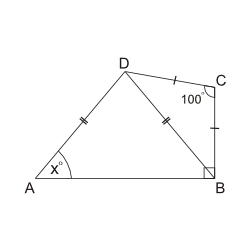We cover every section of the GMAT with in-depth lessons, 5000+ practice questions and realistic practice tests.

## Up to 90+ points GMAT score improvement guarantee

### The best guarantee you’ll find

Our Premium and Ultimate plans guarantee up to 90+ points score increase or your money back.

## Master each section of the test

### Comprehensive GMAT prep

We cover every section of the GMAT with in-depth lessons, 5000+ practice questions and realistic practice tests.

## Schedule-free studying

### Learn on the go

Study whenever and wherever you want with our iOS and Android mobile apps.

# Triangles: IsoscelesIf $$AD = DB$$, $$DC =CB$$, and angle $$ABC = 90º$$ as shown above, then what is the value of $$xº$$?
Correct. [[snippet]] Given that $$CB = CD$$, > $$\displaystyle{\angle CBD = \angle CDB = \frac{180º - 100º}{2} = 40º}$$. Given that angle $$ABC$$ is a right angle, $$\angle DBA = 90º - 40º = 50º$$. Since $$AD = DB$$, $$\angle DAB = \angle DBA$$. Hence, $$xº=50º$$.
Incorrect. [[snippet]]
Incorrect. [[snippet]]
Incorrect. [[snippet]]
Incorrect. [[snippet]]
$$40º$$
$$50º$$
$$65º$$
$$80º$$
$$100º$$